Next: 6.2.2 Second Maxwell Equation Up: 6.2 Lateral Discretization of Previous: 6.2 Lateral Discretization of

## 6.2.1 First Maxwell Equation

The three vector components of the first Maxwell equation take the explicit form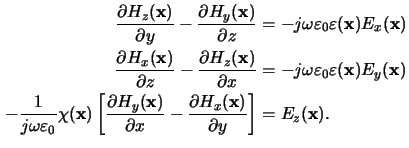Note that for the vertical component we have used the reciprocal permittivity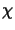(x) =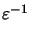(x). Insertion of the Fourier expansions (6.11) and (6.12) and comparison of the coefficients yields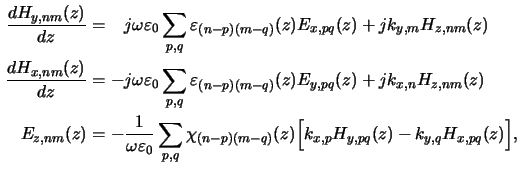whereby the derivatives with respect to the lateral coordinates x and y were transformed into a multiplication of the Fourier coefficients with the respective harmonic frequency jkx, n and jky, m, i.e.,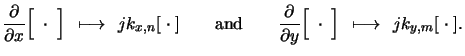(6.13)

The first two equations of (6.15) constitute two coupled ODEs for the lateral magnetic field coefficients Hx, nm(z) and Hy, nm(z), whereas the third equation is an analytical expression for the vertical electric field coefficients Ez, nm(z). The convolution-like relations in the Fourier domain follow from the multiplication in the spatial domain (cp. (6.14) with (6.15)).Next: 6.2.2 Second Maxwell Equation Up: 6.2 Lateral Discretization of Previous: 6.2 Lateral Discretization of
Heinrich Kirchauer, Institute for Microelectronics, TU Vienna
1998-04-17